#Function Repository Resource:

# HardSphereSimulation (2.0.0)current version: 2.1.0 »

Simulate identical unit-mass hard spheres moving in an N-dimensional box

Contributed by: Matt Kafker and Christopher Wolfram
 ResourceFunction["HardSphereSimulation"][assoc] generates a simulation of the motion of a system of identical unit-mass hard spheres with simulation parameters specified by assoc.

## Details

Input assoc must include the following:
 "Positions" initial particle positions "Velocities" initial particle velocities "BoxRadius" half-side-length of simulation box "ParticleRadius" radius of spheres "Steps" number of simulation time steps "dt" duration of a single time step "BoundaryCondition" interaction between particles and container walls "Output" simulation output
The dimensions of vectors in "Positions" and "Velocities" determine the spatial dimension of the system (they can be any positive integer dimension).
The box is centered on the origin.
Possible named boundary conditions include:
 "Reflecting" particle velocities reflect upon contact with container walls "Periodic" particles are translated to opposite side of box upon reaching container walls "Infinite" particles do not alter motion upon reaching container walls
Possible named outputs include:
 "PositionsByTime" list of all particle positions at every time step "VelocitiesByTime" list of all particle velocities at every time step "SpeedsByTime" list of all particle speeds at every time step "TrajectoriesByTime" list of all particle positions and velocities at every time step "PositionsByParticle" list of positions over time for each particle "VelocitiesByParticle" list of velocities over time for each particle "SpeedsByParticle" list of speeds over time for each particle "TrajectoriesByParticle" list of positions and velocities over time for each particle "Collisions" list of particle-particle collisions "All" list containing “TrajectoriesByTime” output as the first entry, and “Collisions” as the second "Visualize" Graphics object with a Manipulate slider for visualizing the time-evolution of the system in one, two, or three spatial dimensions "KineticEnergy" list of total kinetic energy at every time step "FinalFrame" Graphics object depicting the state of the system at the final time step "CausalGraph" Graph object with nodes representing collisions and directed edges indicating that one particle was contiguously involved in two collisions
Each collision takes the form {particlei,particlej,time of collision}.
The option "Random" may be given for pos, in which case particle positions are chosen at random within the box.

## Examples

### Basic Examples (2)

Find the position of two hard disks over five time steps:

 In:=Out=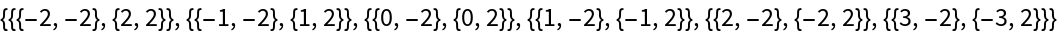Get the velocities over time:

 In:=Out=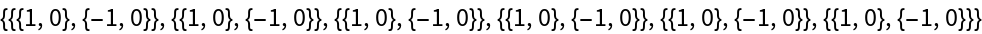Get the speeds over time:

 In:=Out=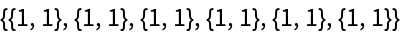Get the trajectories over time:

 In:=Out=Return the positions grouped by particle:

 In:=Out=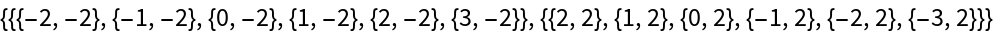Get the velocities by particle:

 In:=Out=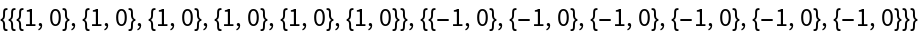Get the speeds by particle:

 In:=Out=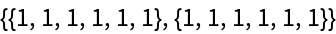Get the trajectories by particle:

 In:=Out=### Scope (5)

Use HardSphereSimulation to visualize many hard spheres moving in a box:

 In:=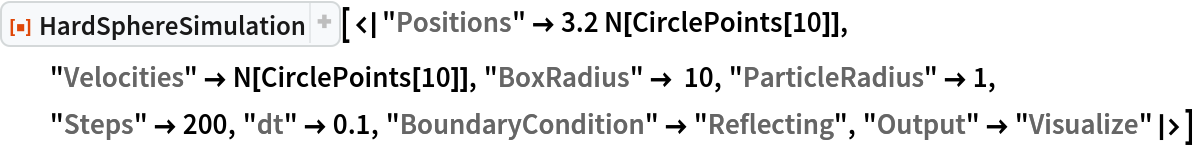Out=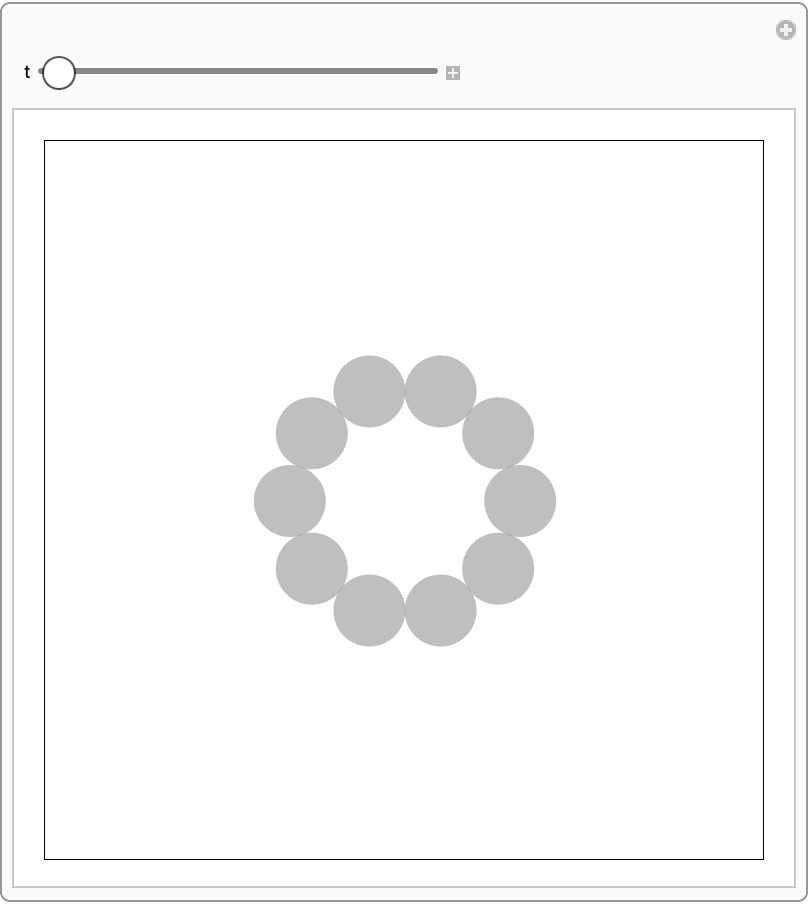Use "Random" to generate random initial positions in the box:

 In:=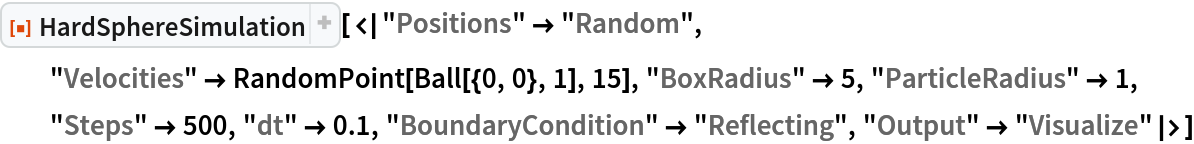Out=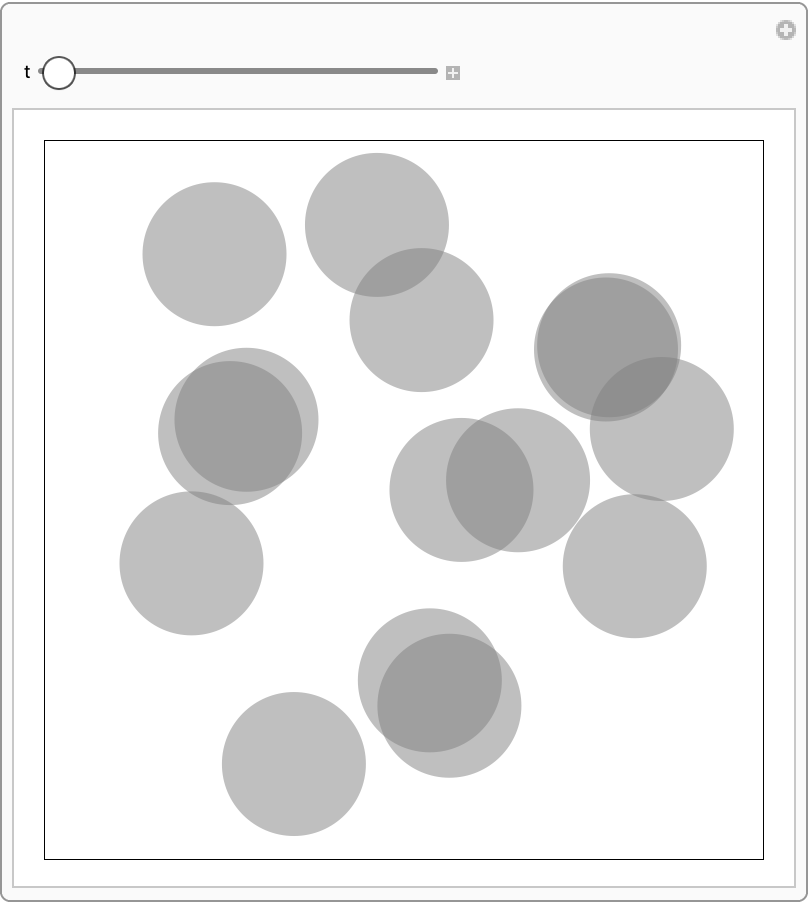Visualization works in 1D and 3D as well:

 In:=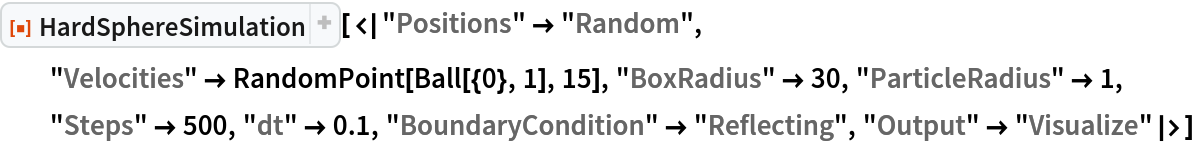Out=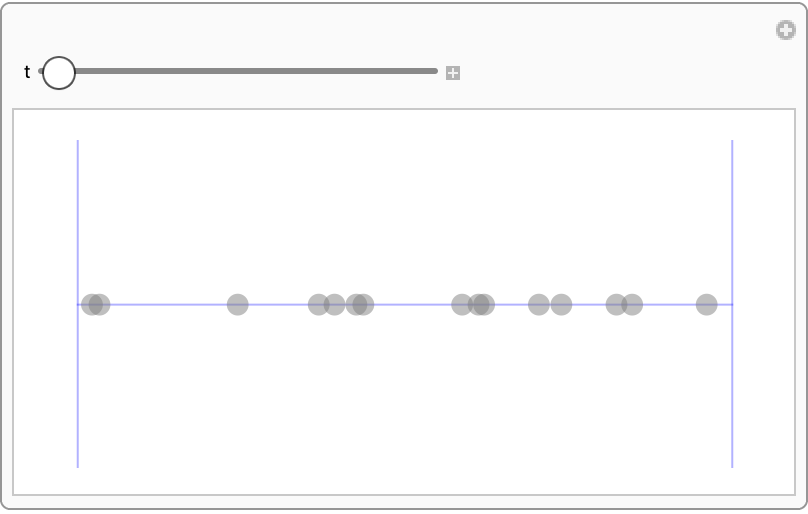In:=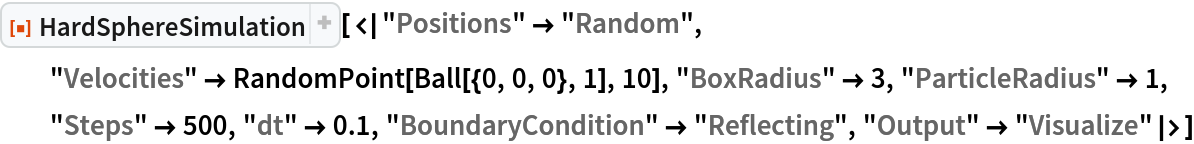Out=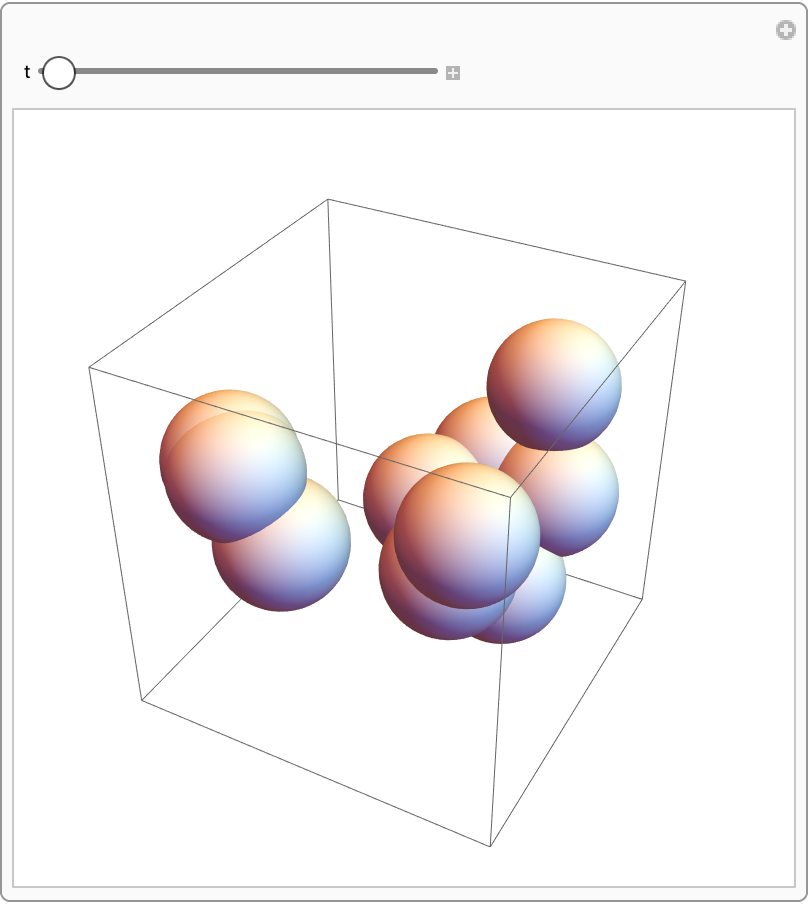Periodic boundary conditions identify opposite walls of the box with one another:

 In:=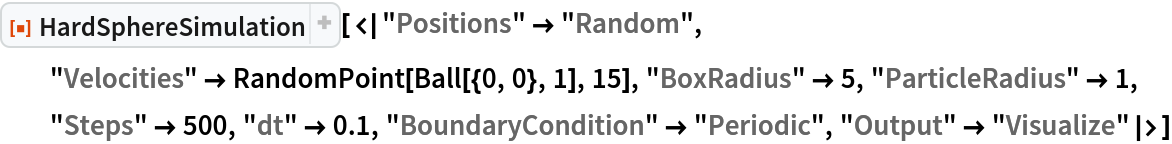Out=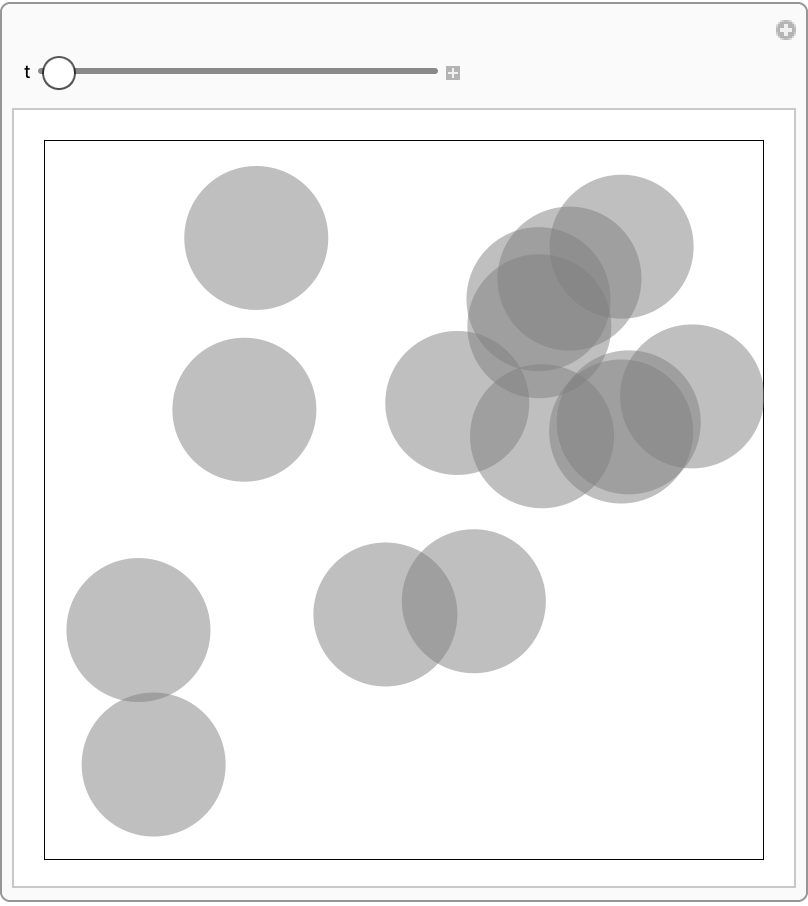Infinite boundary conditions eliminate particle-wall interactions altogether:

 In:=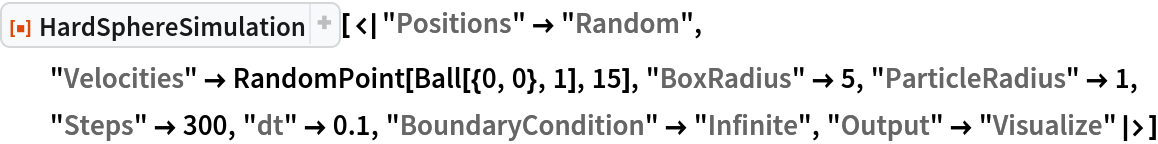Out=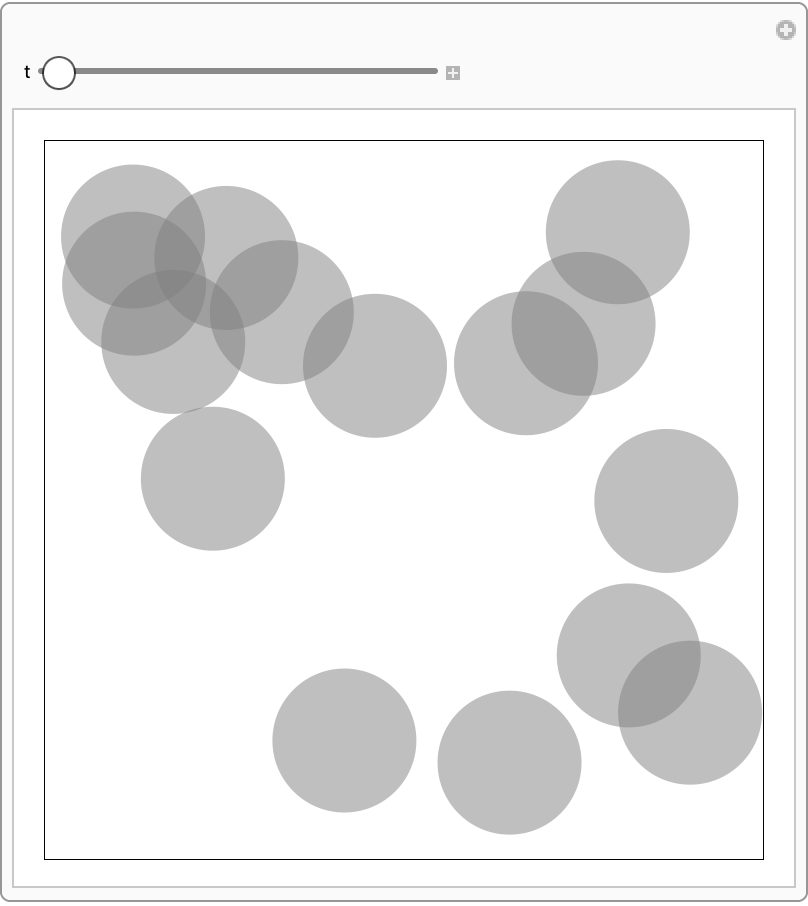### Applications (3)

Particle speeds should obey Maxwell-Boltzmann distribution:

 In:=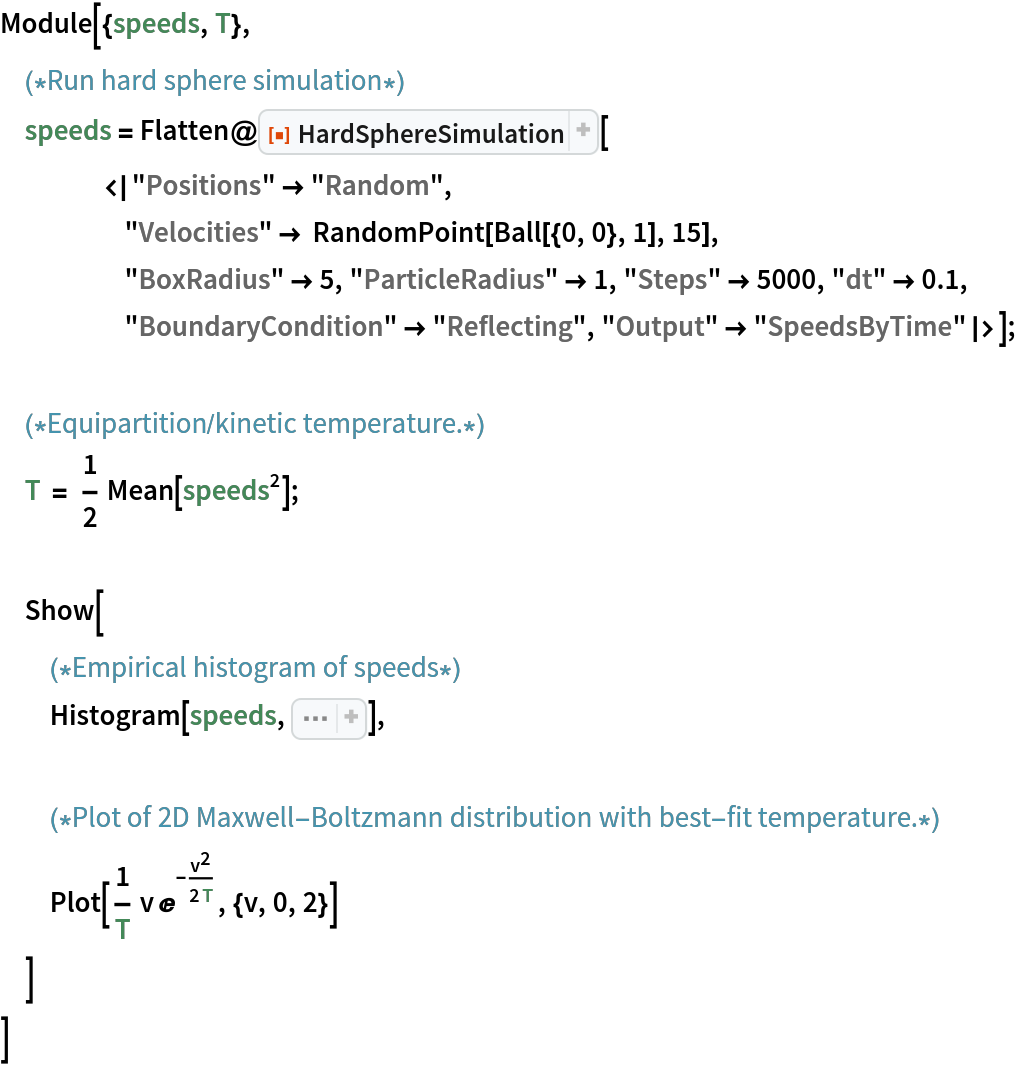Out=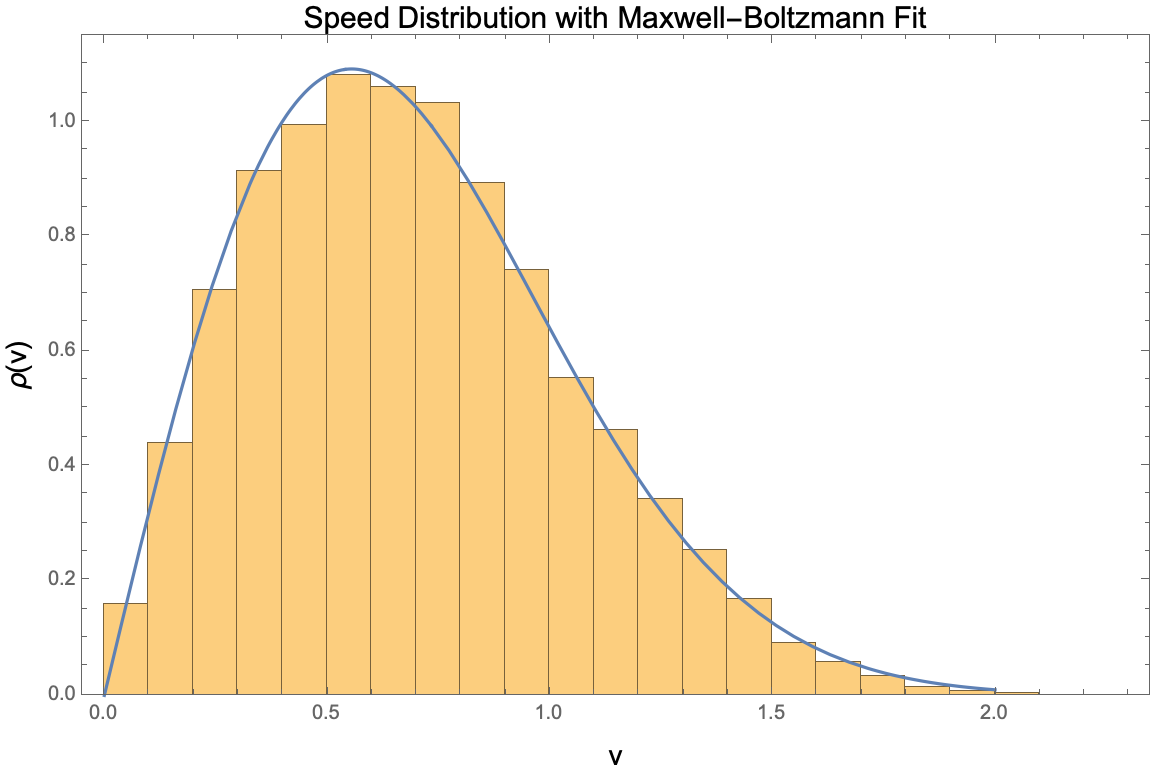Extract a collision list from the simulation, where collisions take the form {particle1,particle2,time of collision}:

 In:=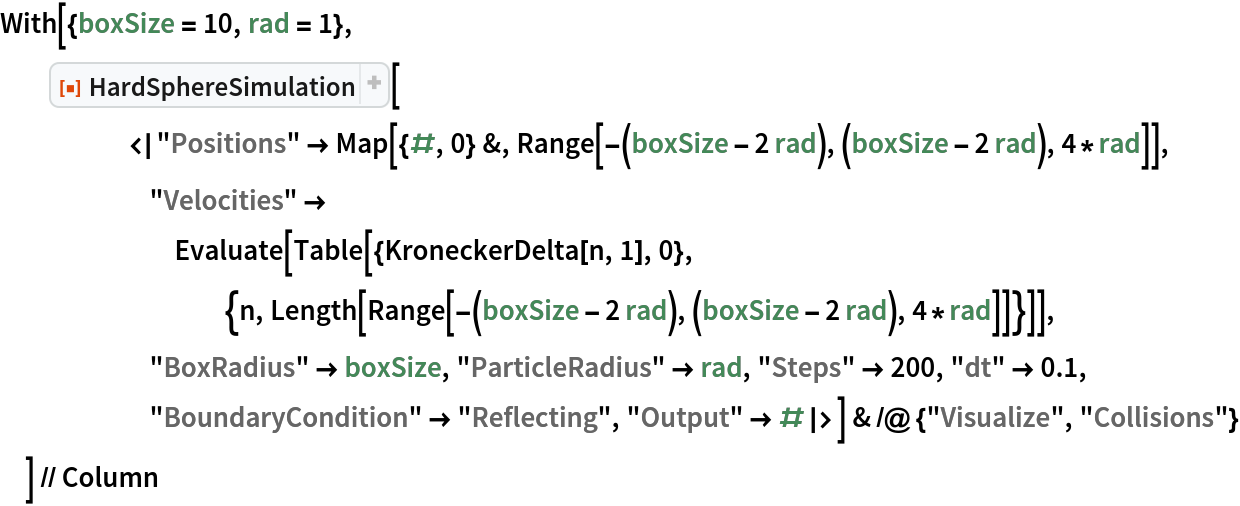Out=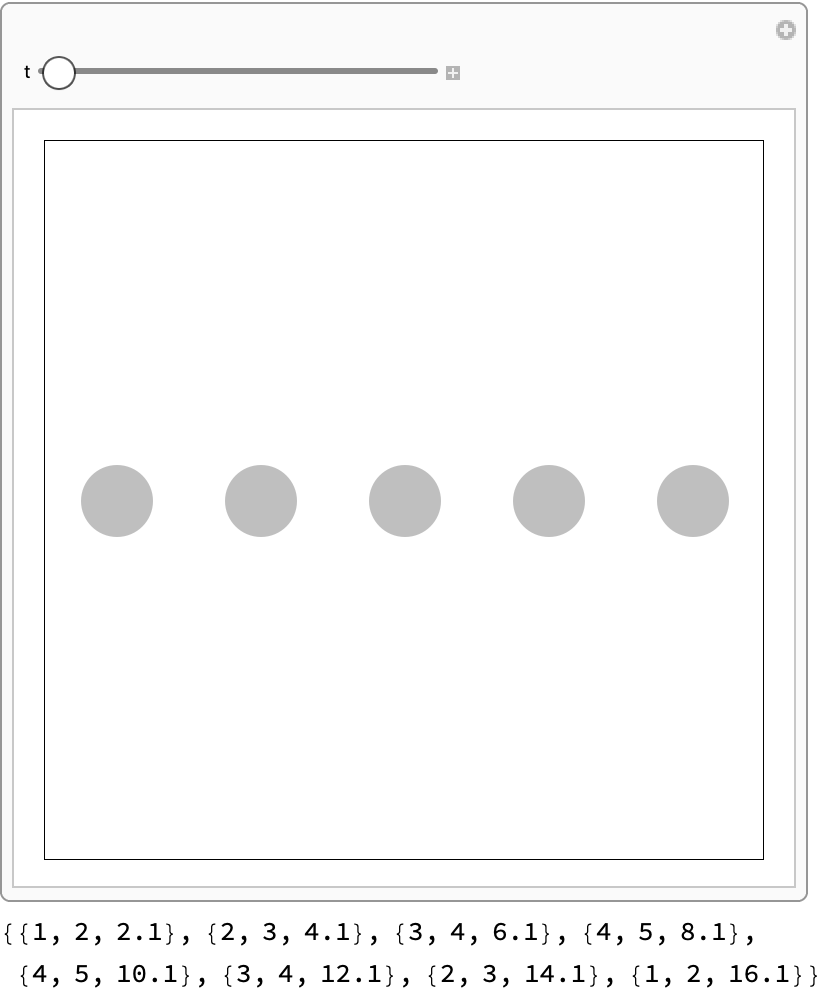From particle-particle collisions, construct the causal graph:

 In:=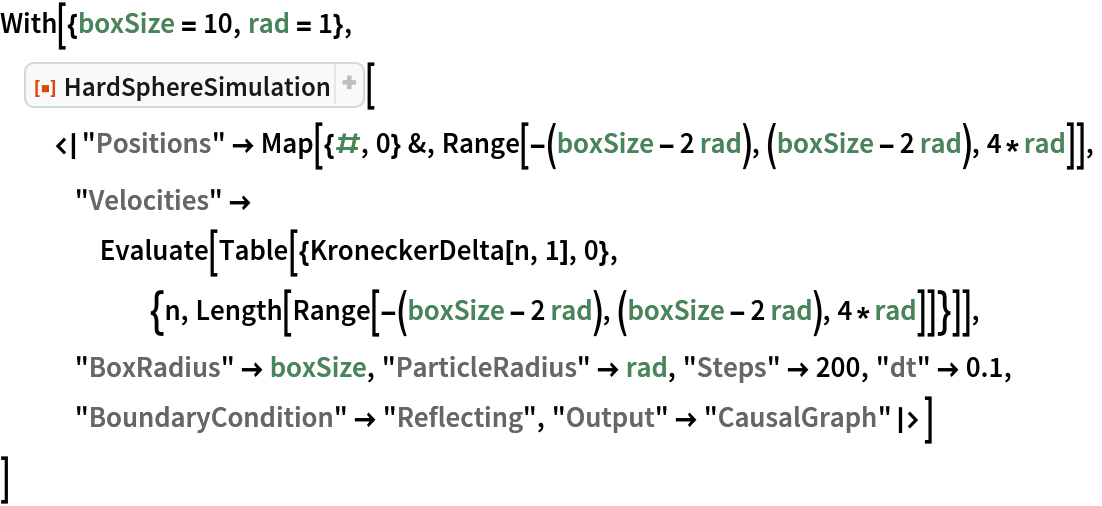Out=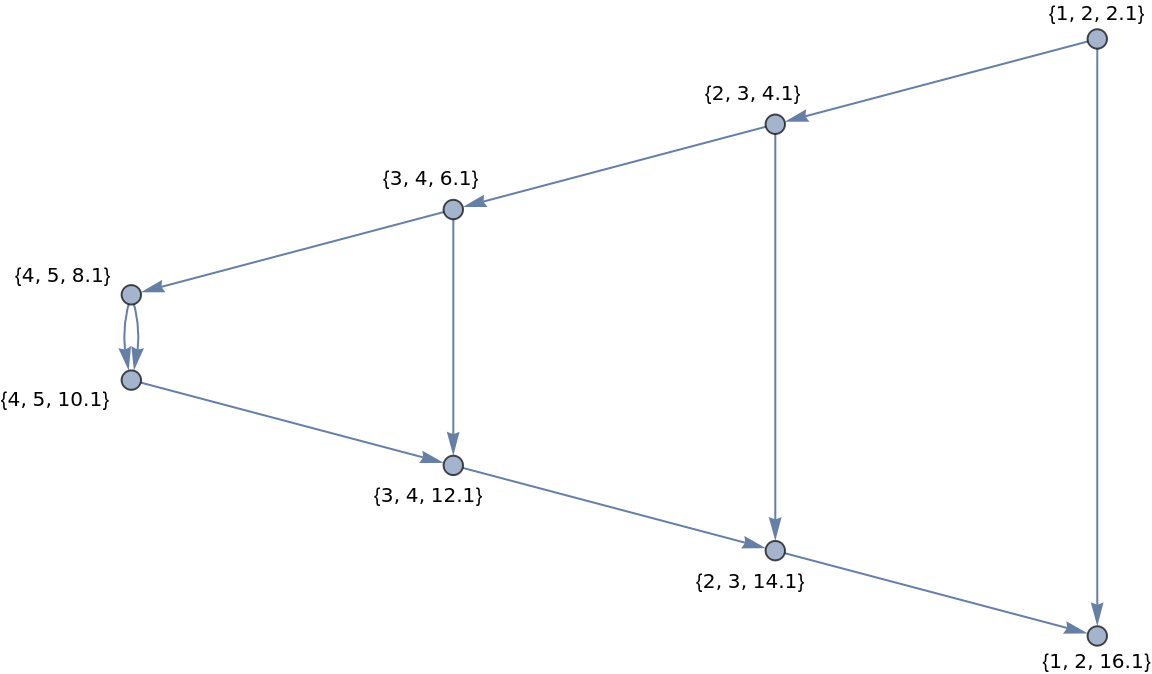Construct a causal graph for a disordered system (ensuring initial positions do not overlap to avoid spurious collisions):

 In:=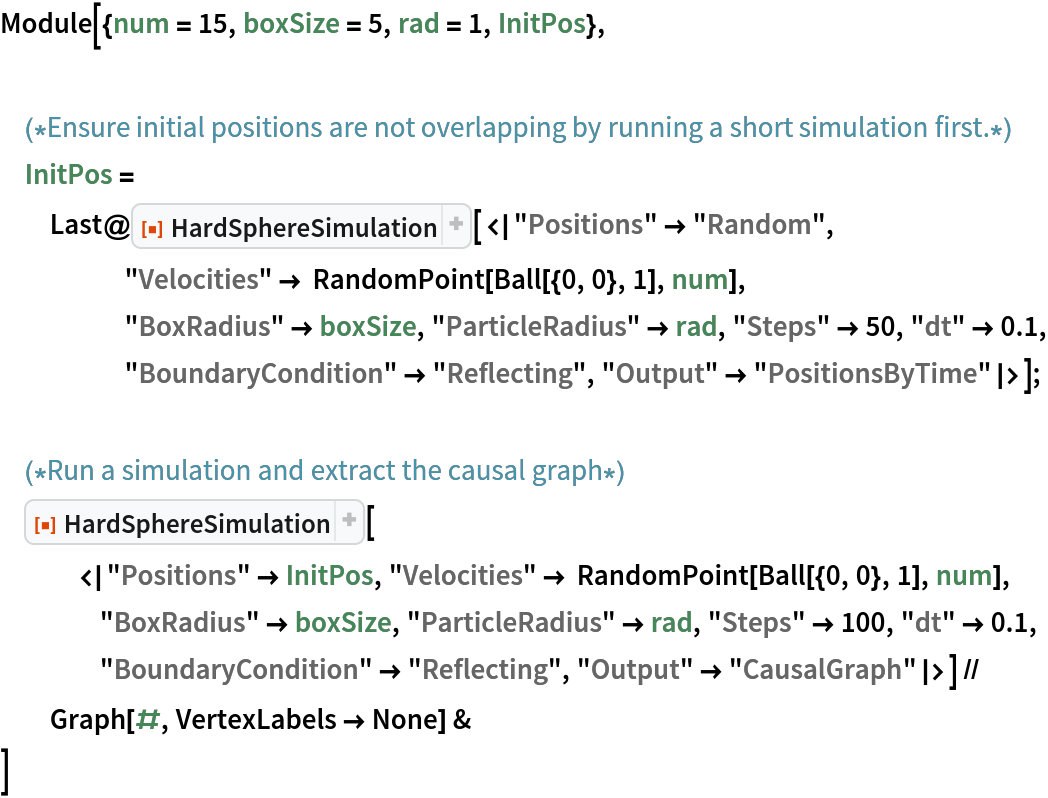Out=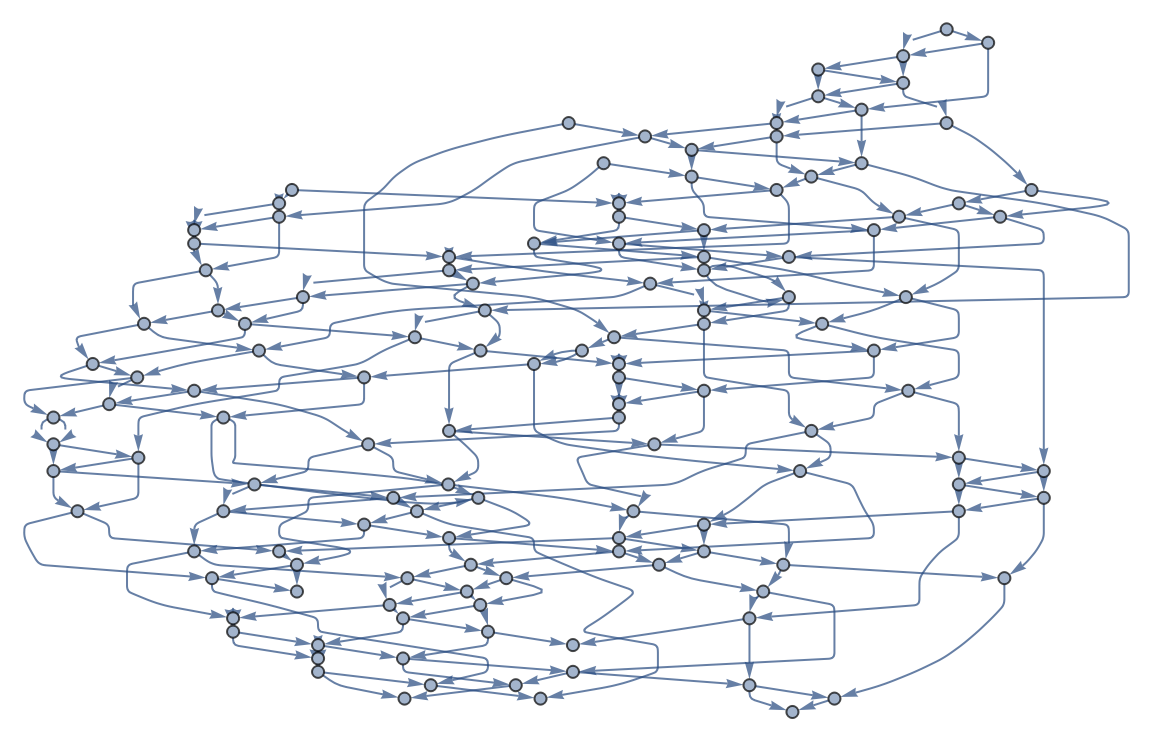As the nodes of the causal graph represent collisions between two particles and the edges represent individual particles, we expect all nodes to have indegree and outdegree 2, besides from the graph boundary (i.e., the beginning and end of the simulation):

 In:=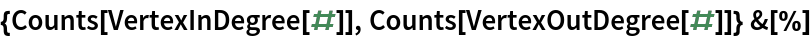Out=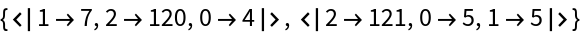### Properties and Relations (2)

Total kinetic energy of spheres should be conserved in time. Show that the error stays within machine precision:

 In:=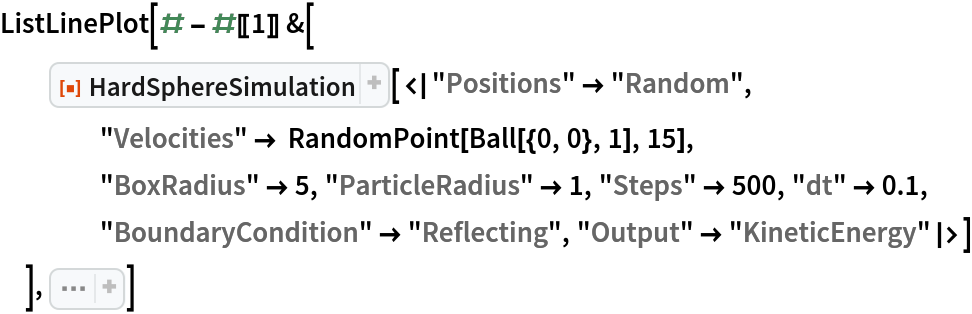Out=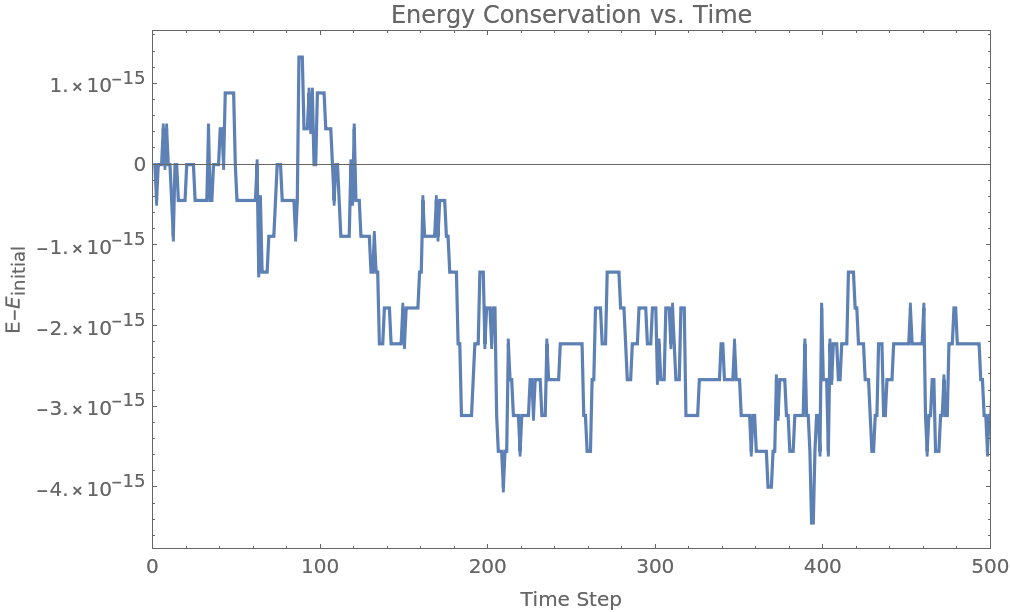Momentum should be conserved during collisions between particles:

 In:=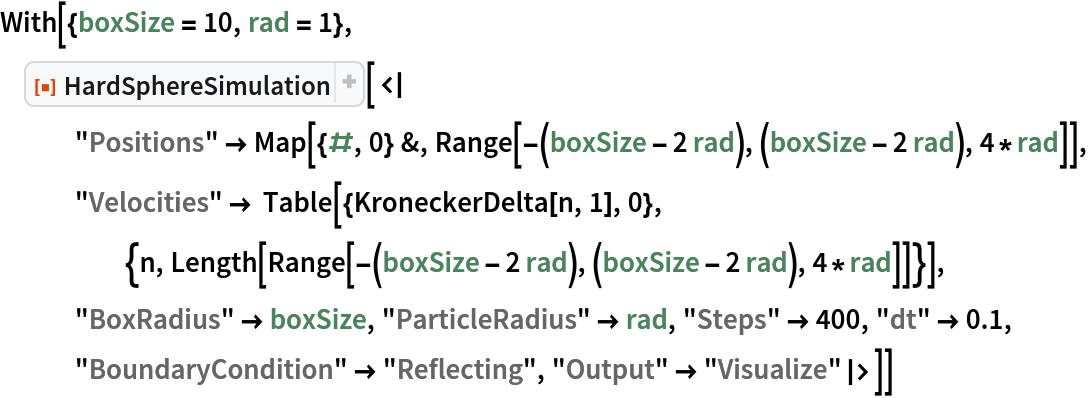Out=### Possible Issues (1)

Particle overlaps are resolved pairwise, so situations involving multiple overlapping spheres may lead to unexpected results (such as a disruption of symmetry):

 In:=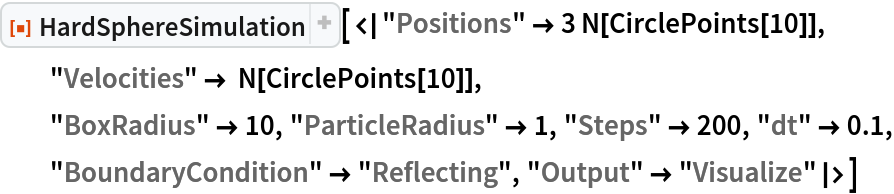Out=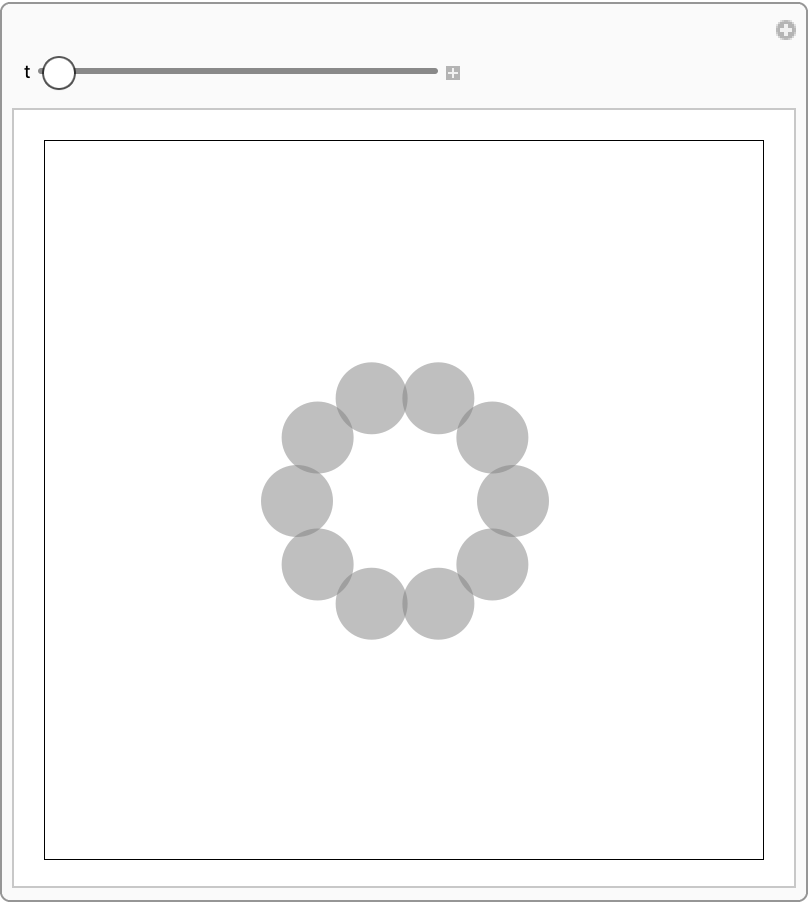### Neat Examples (2)

 In:=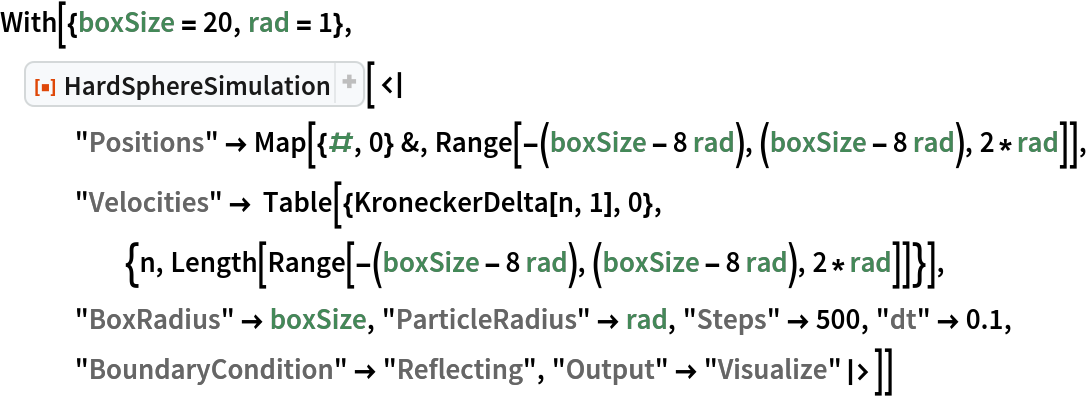Out=Use HardSphereSimulation to simulate a simplified game of pool:

 In:=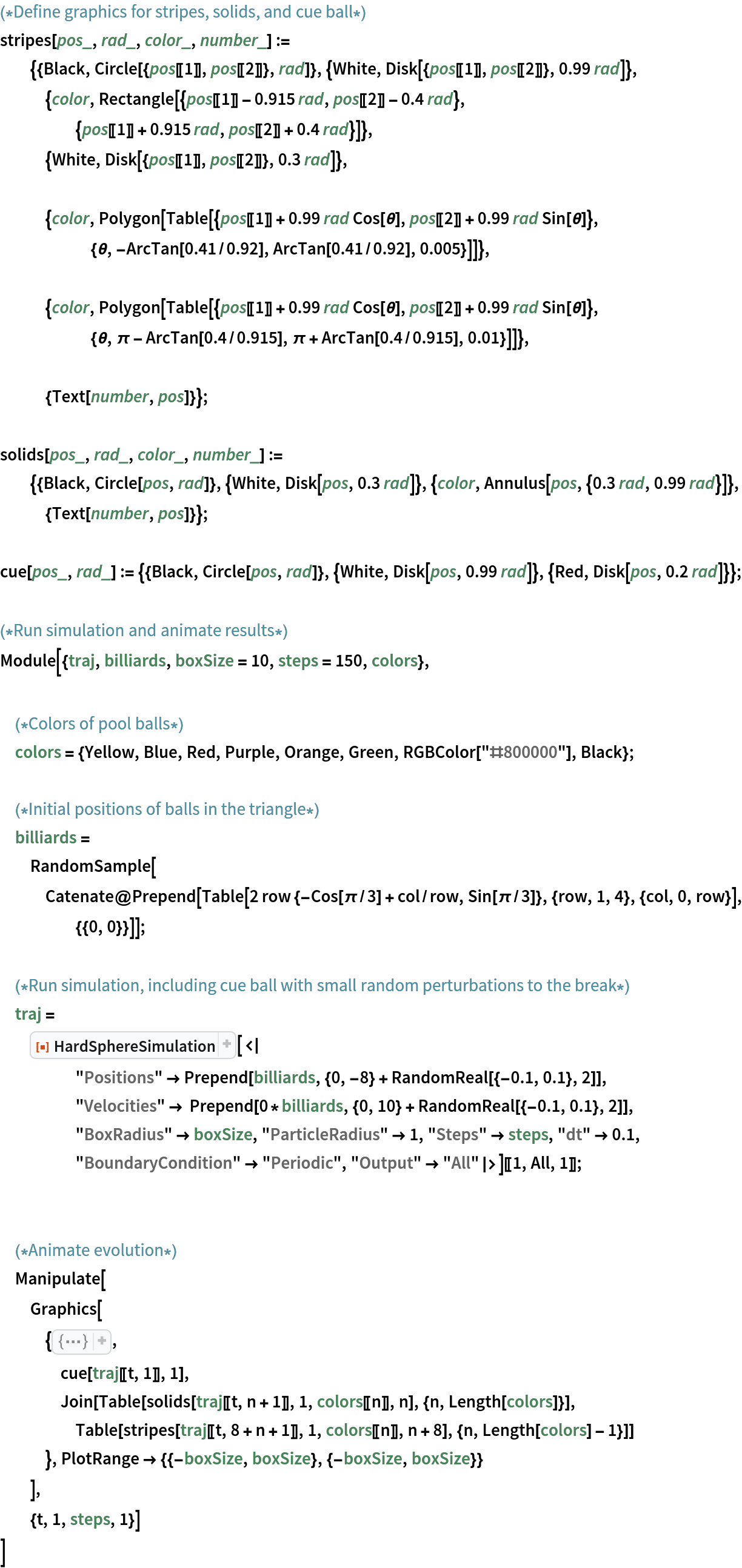Out=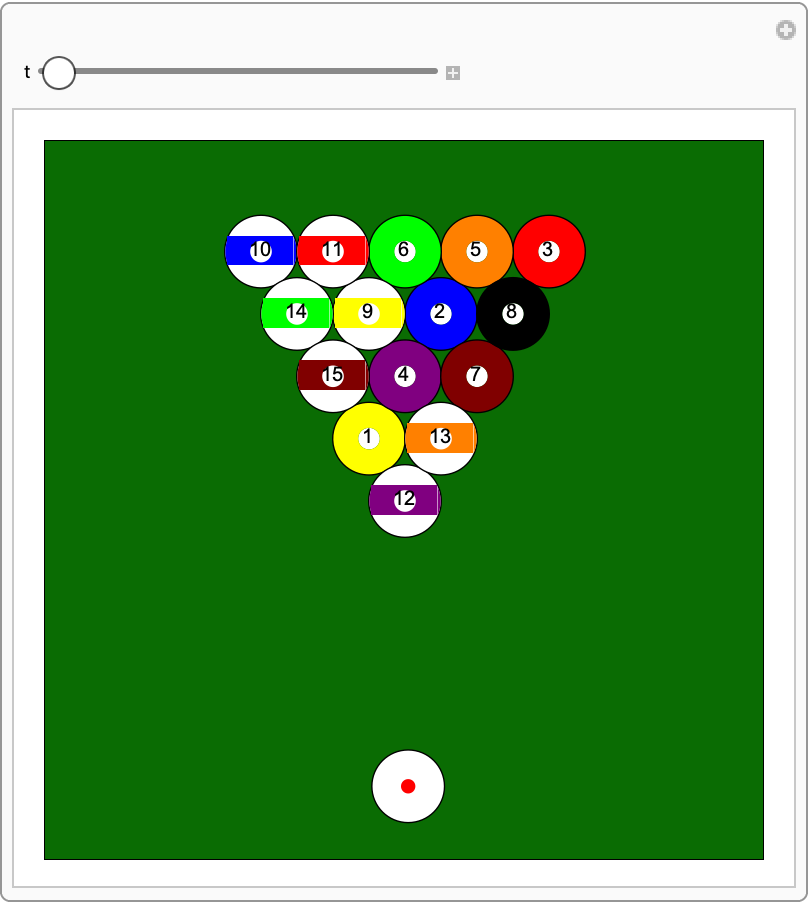## Publisher

Wolfram Summer School

## Version History

• 2.1.0 – 30 December 2022
• 2.0.1 – 14 October 2022
• 2.0.0 – 28 September 2022
• 1.0.0 – 16 February 2021